# 7th grade (12y) - math problems

Mathematical word problems allow you to practice your mathematics knowledge in everyday life tasks. Word problems train to understand, translate into the mathematical language (e.g., equations), solve it, and check the accuracy and solution discussion.
Choose a topic you want to calculate and improve in.

### From our database of math word problems and examples we offer:

• Divide an isosceles triangleHow to divide an isosceles triangle into two parts with equal contents perpendicular to the axis of symmetry (into a trapezoid and a triangle)?Find the roots of the quadratic equation: 3x2-4x + (-4) = 0.
• Vertices of a right triangleShow that the points D(2,1), E(4,0), F(5,7) are vertices of a right triangle.
• LatheFrom the cube of edge, 37 cm was a lathed maximum cylinder. What percentage of the cube is left as waste after lathed?
• Controller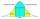Output Controller of the company in the control of 50 randomly selected products found that 37 of them had no defects, 8 has only one flaw, three had two defects, and two products had three defects. Determine the standard deviation and coefficient of vari
• Holiday SMS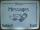Kucera and Malek start from the holiday together. They agreed that if they be 100 km apart, will send a SMS. Kucera traveling at 60 km/h., Malek 90 km/h. They started at 14 hours PM. At what time they send a message?
• James 2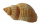James collected 24 seashells on the seashore to be shared with his 2 friends. What fractional part of the seashells will each one get if it is distributed evenly? ​
• ForcesIn point O acts three orthogonal forces: F1 = 20 N, F2 = 7 N, and F3 = 19 N. Determine the resultant of F and the angles between F and forces F1, F2, and F3.
• Evaluate fractionsThe difference  of 5 1/2 and 2/3 is added to the product of 5/6 and 1/2
• StudentsStudents solve word problems. They received 3 points for each correctly solved task. Four points were deducted for a task that was not solved or incorrectly solved. Tomas solved a total of 15 tasks and scored 24 points. How many tasks did he solve correct
• TianaTiana is buying party favors for the guests at her 12th birthday party. Each party favor costs \$2.39. If she buys 15 party favors, how much money does she spend?
• Circle arcCalculate the circular arc area in m2 where the diameter is 263 dm, and a central angle is 40°. Result round to three decimal places.
• Normal distribution GPA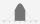The average GPA is 2.78 with a standard deviation of 4.5. What are students in the bottom the 20% having what GPA?
• Homeless Dezider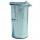Homeless Dežko has 9 coins in jacket: Calculate the value of its assets and calculate how many bottles of wine for 0.55 EUR can he buy.
• Simple equationSolve the following simple equation: 2. (4x + 3) = 2-5. (1-x)
• English and math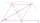1/5 of a class study English, and 3/5 study mathematics. If the class consist of 80 students, how many students study English and mathematics in the class?
• Right triangleCalculate the missing side b and interior angles, perimeter, and area of ​​a right triangle if a=10 cm and hypotenuse c = 16 cm.